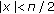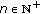# Sharpening the Becker-Stark Inequalities

## Abstract

In this paper, we establish a general refinement of the Becker-Stark inequalities by using the power series expansion of the tangent function via Bernoulli numbers and the property of a function involving Riemann's zeta one.

## 1. Introduction

Steckin  (or see Mitrinovic [2, 3.4.19, page 246]) gives us a result as follows.

Theorem 1.1 (see [1, Lemma]).

If, then(11)

Later, Becker and Stark  (or see Kuang [4, 5.1.102, page 248]) obtain the following two-sided rational approximation for.

Theorem 1.2.

Let, then(12)

Furthermore,andare the best constants in (1.2).

In fact, we can obtain the following further results.

Theorem 1.3.

Let, then(13)

Furthermore,andare the best constants in (1.3).

In this paper, in the form of (1.2) and (1.3) we shall show a general refinement of the Becker-Stark inequalities as follows.

Theorem 1.4.

Let, and letbe a natural number. Then(14)

holds, where, and(15)

whereare the even-indexed Bernoulli numbers.

Furthermore,andare the best constants in (1.4).

## 2. Four Lemmas

Lemma 2.1.

The functionis decreasing, whereis Riemann's zeta function.

Proof.is equivalent to the function, which is decreasing.

Lemma 2.2 (see [5, Theorem]).

Letbe Riemann's zeta function andthe even-indexed Bernoulli numbers. Then(21)

Lemma 2.3 (see [6, 1.3.1.4 (1.3)]).

Let. Then(22)

Lemma 2.4.

Letand. Then, where(23)

Proof.

By Lemma 2.3, we have(24)

Sinceis decreasing by Lemma 2.1, it follows that(25)

From Lemma 2.2, we get(26)

which implies thatfor.

## 3. Proofs of Theorems

Proof of Theorem 1.4.

Let(31)

Then(32)

By Lemma 2.4, we havefor, andis decreasing on.

At the same time,=by (3.1), andby (3.2), soandare the best constants in (1.4).

Proof of Theorem 1.3.

Letin Theorem 1.4; we obtain thatand. Then the proof of Theorem 1.3 is complete.

## References

1. Steckin SB: Some remarks on trigonometric polynomials. Uspekhi Matematicheskikh Nauk 1955, 10(1(63)):159–166.

2. Mitrinovic DS: Analytic Inequalities. Springer, New York, NY, USA; 1970:xii+400.

3. Becker M, Strak EL: On a hierarchy of quolynomial inequalities for tanx. University of Beograd Publikacije Elektrotehnicki Fakultet. Serija Matematika i fizika 1978, (602–633):133–138.

4. Kuang JC: Applied Inequalities. 3rd edition. Shangdong Science and Technology Press, Jinan City, China; 2004.

5. Scharlau W, Opolka H: From Fermat to Minkowski, Undergraduate Texts in Mathematics. Springer, New York, NY, USA; 1985:xi+184.

6. Jeffrey A: Handbook of Mathematical Formulas and Integrals. 3rd edition. Elsevier Academic Press, San Diego, Calif, USA; 2004:xxvi+453.

## Author information

Authors

### Corresponding author

Correspondence to Ling Zhu.

## Rights and permissions

Reprints and Permissions

Zhu, L., Hua, J. Sharpening the Becker-Stark Inequalities. J Inequal Appl 2010, 931275 (2010). https://doi.org/10.1155/2010/931275

• Accepted:

• Published:

• DOI: https://doi.org/10.1155/2010/931275

### Keywords

• Power Series
• Natural Number
• Series Expansion
• Zeta Function
• Power Series Expansion